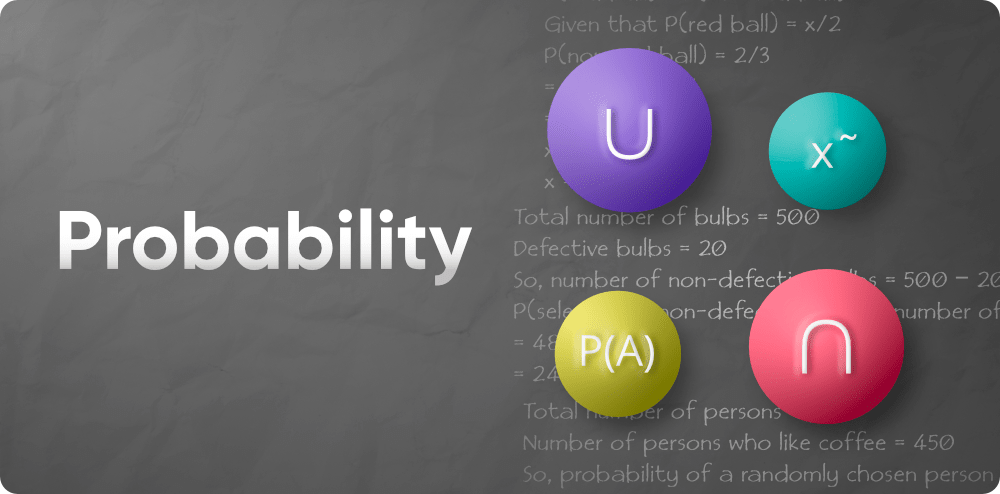Skip to content
Related Articles
Probability in Maths
• Last Updated : 03 Jun, 2021

Probability denotes the possibility of anything happening. It is a mathematical branch that deals with the occurrence of a random event. The value ranges from zero to one. Probability has been introduced in mathematics to estimate the likelihood of events occurring. Probability is described as the degree to which something is expected to occur. This is the fundamental probability principle, which is often found in the probability distribution, in which you can hear about the possible results of a random experiment. To determine the likelihood of a particular occurrence occurring, we must first determine the total number of potential outcomes.Probability is a calculation of how likely an incident is to occur. Many events are impossible to forecast with absolute certainty. Using it, we can only estimate the likelihood of an occurrence occurring, i.e. how probable it is to occur. The probability will vary from 0 to 1, with 0 indicating an unlikely occurrence and 1 indicating a certain event.

### Theoretical Probability

It is focused on the likelihood of anything occurring. The reasoning behind probability is the foundation of scientific probability. If a coin is flipped, the statistical chance of having a head is 1/2. An event’s statistical chance is the chance that the occurrence will occur. It is determined by dividing the number of desirable outcomes by the total number of outcomes is thus termed as theoretical probability and can be learned in the following articles:

### Experimental Probability

It is founded on the results of an experiment. The experimental chance can be determined by dividing the total number of trials by the number of potential outcomes. For example, if a coin is flipped ten times and heads are reported six times, the experimental chance of heads is 6/10, or 3/5. Let’s discuss the experimental probability in the following articles:

### Axiomatic Probability

A collection of laws or axioms that apply to all forms is established in axiomatic probability. Kolmogorov developed these axioms, which are known as Kolmogorov’s three axioms. The axiomatic approach to probability quantifies the likelihood of events occurring or not occurring. This definition is covered in detail in the axiomatic probability articles, which include Kolmogorov’s three laws (axioms) as well as numerous examples. The chance of an event or result happening depending on the existence of a prior event or outcome is known as conditional probability. Following are the articles based on the axiomatic probability:

My Personal Notes arrow_drop_up